SBOS618I December   2013  – May 2018

PRODUCTION DATA.

1. Features
2. Applications
3. Description
1.     Device Images
4. Revision History
5. Device Comparison
6. Pin Configuration and Functions
7. Specifications
8. Detailed Description
1. 8.1 Overview
2. 8.2 Functional Block Diagram
3. 8.3 Feature Description
4. 8.4 Device Functional Modes
9. Applications and Implementation
1. 9.1 Application Information
2. 9.2 Typical Applications
10. 10Power-Supply Recommendations
11. 11Layout
12. 12Device and Documentation Support
1. 12.1 Device Support
1. 12.1.1 Development Support
2. 12.2 Documentation Support
4. 12.4 Community Resources
6. 12.6 Electrostatic Discharge Caution
7. 12.7 Glossary
13. 13Mechanical, Packaging, and Orderable Information

• D|8
• DRG|8
• DGK|8
• D|8
• DRG|8
• DGK|8

### 7.9 Typical Characteristics

At VS = ±18 V, VCM = VS / 2, RLOAD = 10 kΩ connected to VS / 2, and CL = 100 pF, unless otherwise noted.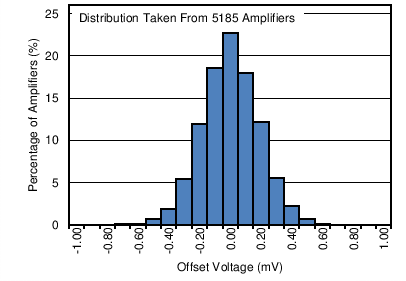Figure 1. Offset Voltage Production Distribution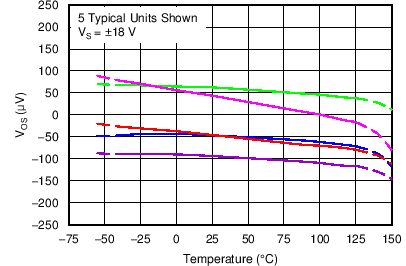Figure 3. Offset Voltage vs Temperature
(VS = ±18 V)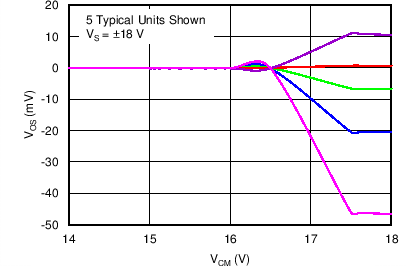Figure 5. Offset Voltage vs Common-Mode Voltage
(Upper Stage)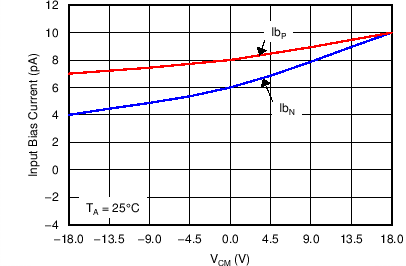Figure 7. Input Bias Current vs Common-Mode Voltage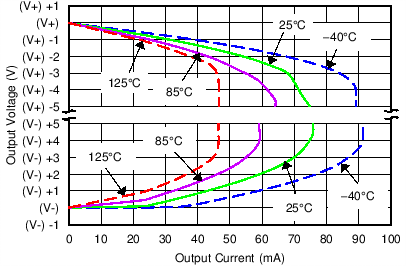Figure 9. Output Voltage Swing vs Output Current (Maximum Supply)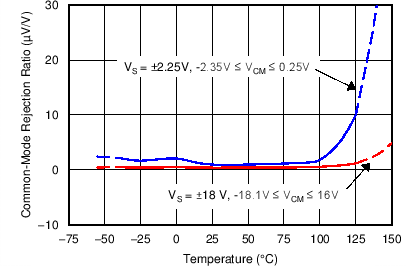Figure 11. CMRR vs TemperatureFigure 13. 0.1-Hz to 10-Hz NoiseFigure 15. THD+N Ratio vs FrequencyFigure 17. Quiescent Current vs Temperature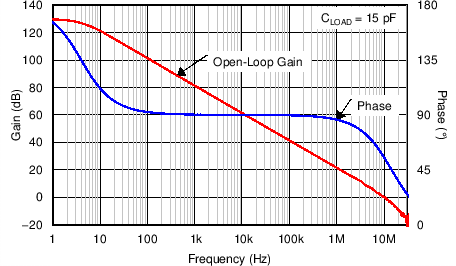Figure 19. Open-Loop Gain and Phase vs Frequency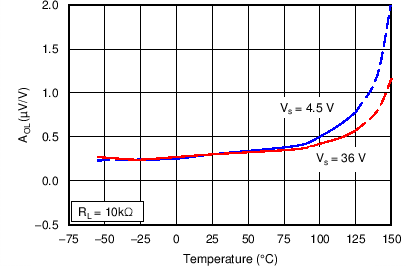Figure 21. Open-Loop Gain vs Temperature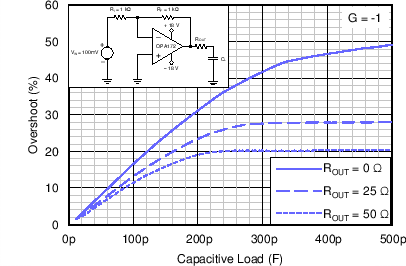Figure 23. Small-Signal Overshoot vs Capacitive Load
(100-mV Output Step)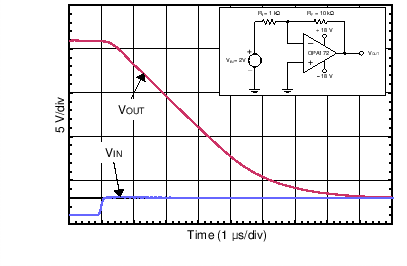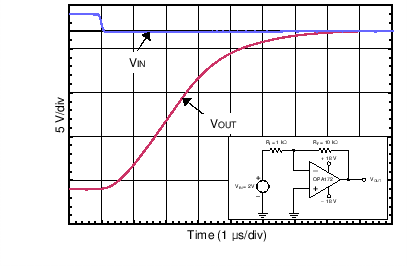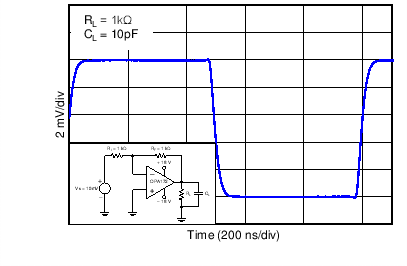Figure 29. Small-Signal Step Response (10 mV, G = –1)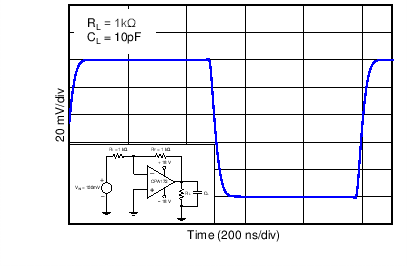Figure 31. Small-Signal Step Response (100 mV, G = –1)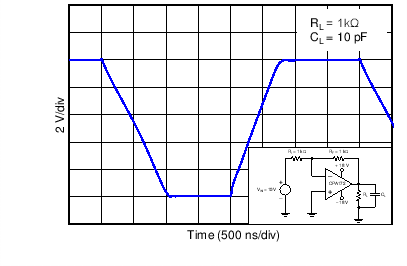Figure 33. Large-Signal Step Response (10 V, G = –1)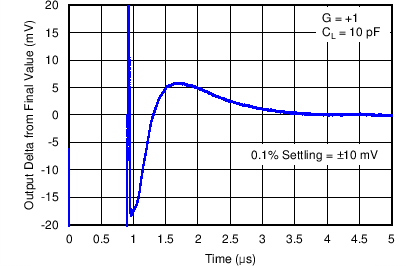Figure 35. Large-Signal Settling Time (10-V Positive Step)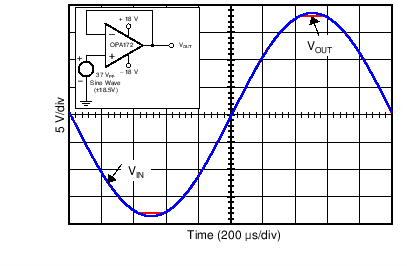Figure 37. No Phase Reversal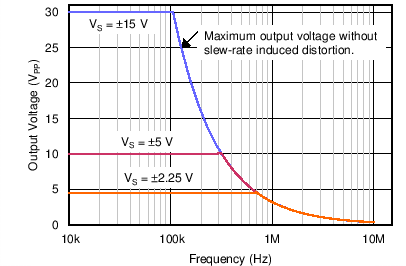Figure 39. Maximum Output Voltage vs Frequency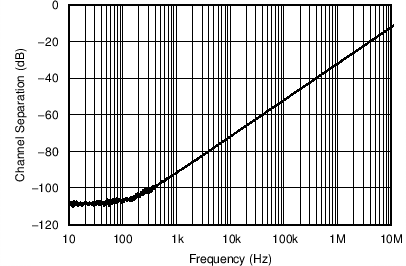Figure 41. Channel Separation vs Frequency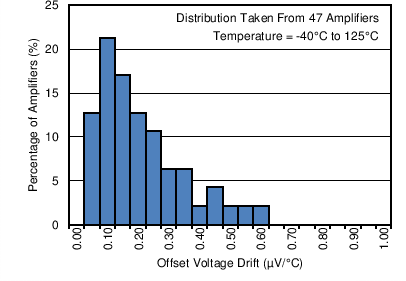Figure 2. Offset Voltage Drift Production Distribution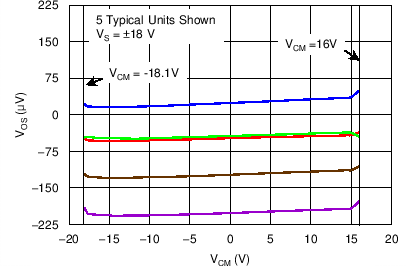Figure 4. Offset Voltage vs Common-Mode Voltage
(VS = ±18 V)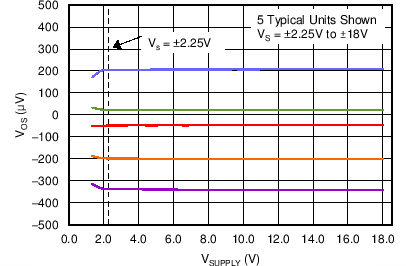Figure 6. Offset Voltage vs Power Supply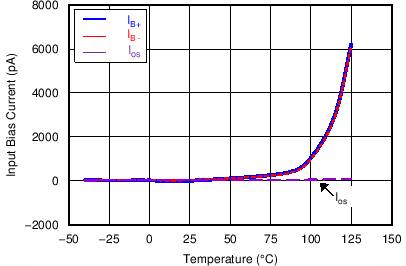Figure 8. Input Bias Current vs Temperature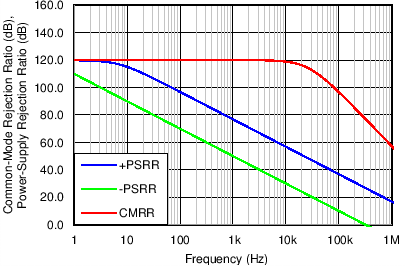Figure 10. CMRR and PSRR vs Frequency
(Referred-To-Input)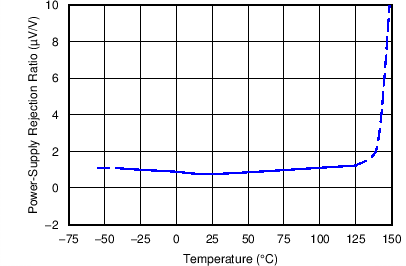Figure 12. PSRR vs Temperature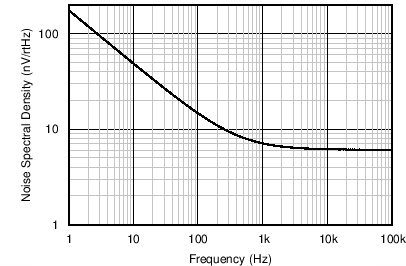Figure 14. Input Voltage Noise Spectral Density vs Frequency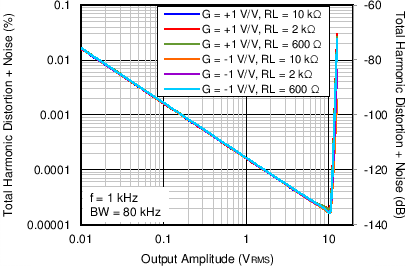Figure 16. THD+N vs Output AmplitudeFigure 18. Quiescent Current vs Supply Voltage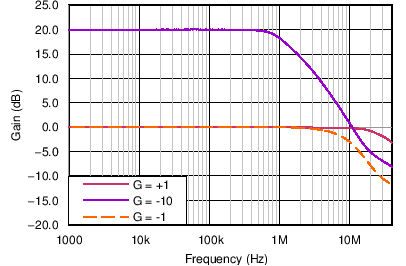Figure 20. Closed-Loop Gain vs Frequency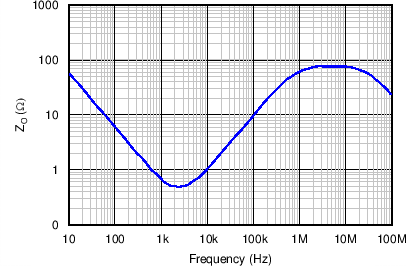Figure 22. Open-Loop Output Impedance vs Frequency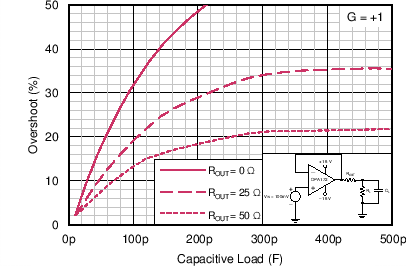Figure 24. Small-Signal Overshoot vs Capacitive Load
(100-mV Output Step)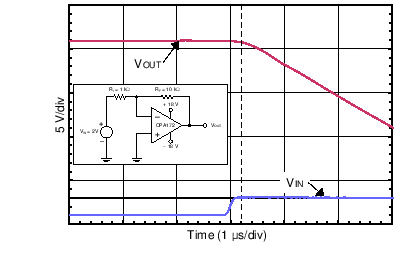Figure 26. Positive Overload Recovery (Zoomed In)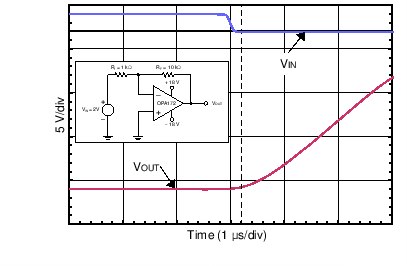Figure 28. Negative Overload Recovery (Zoomed In)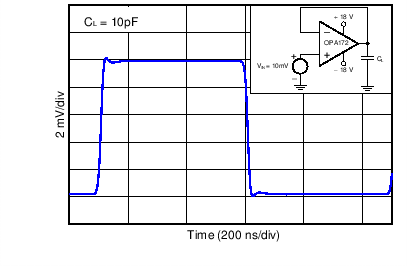Figure 30. Small-Signal Step Response (10 mV, G = +1)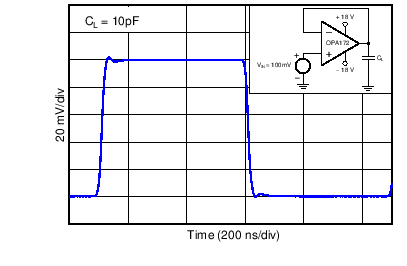Figure 32. Small-Signal Step Response (100 mV, G = +1)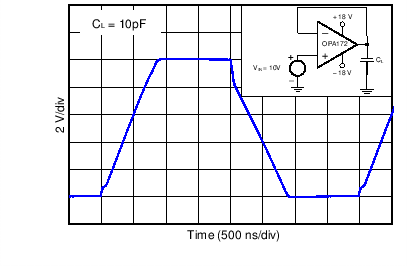Figure 34. Large-Signal Step Response (10 V, G = +1)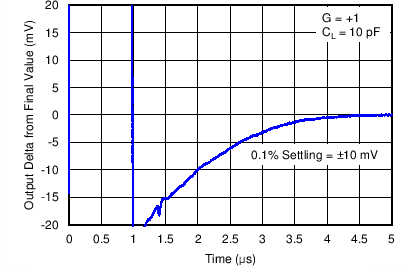Figure 36. Large-Signal Settling Time (10-V Negative Step)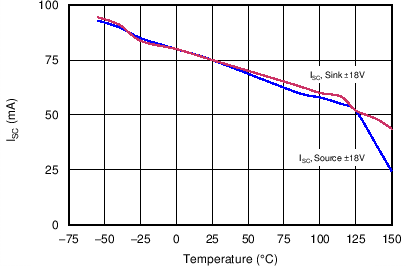Figure 38. Short-Circuit Current vs TemperatureFigure 40. EMIRR vs Frequency